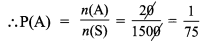Samacheer Kalvi 9th Maths Solutions Chapter 9 Probability Ex 9.2

Tamilnadu Samacheer Kalvi 9th Maths Solutions Chapter 9 Probability Ex 9.2

Question 1.
A company manufactures 10000 Laptops in 6 months. Out of which 25 of them are found to be defective. When you choose one Laptop from the manufactured, what is the probability that selected Laptop is a good one.
Solution:
Total n(S) = 10,000
Defective n( A) = 25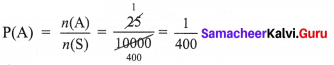No. of good laptops = 1000 – 25
n(B) = 9975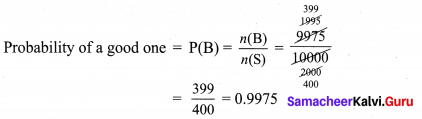Question 2.
In a survey of 400 youngsters aged 16-20 years, it was found that 191 have their voter ID card. If a youngster is selected at random, find the probability that the youngster does not have their voter ID card.
Solution:
No. of youngsters n(S) = 400
No. of youngsters having voter id n(A) = 191
No. of youngsters do not have their voter id n(B) = 400 – 191 = 209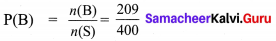Question 3.
The probability of guessing the correct answer to a certain question is $$\frac{x}{3}$$. If the probability of not guessing the correct answer is $$\frac{x}{5}$$, then find the value of x.
Solution: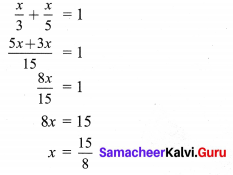Question 4.
If a probability of a player winning a particular tennis match is 0.72. What is the probability of the player loosing the match?
Solution:
P(A) = 0.72
P(A’) = 1 – 0.72 = 0.28

Question 5.
1500 families were surveyed and following data was recorded about their maids at homes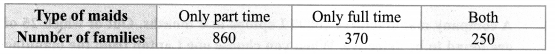A family is selected at random. Find the probability that the family selected has
(i) Both types of maids
(ii) Part time maids
(iii) No maids
Solution:
n(S) = 1500 (Total families)
n(A) = 860 (Part time maids)
n(B) = 370 (Only full time)
n(A∩B) = 250 (Both)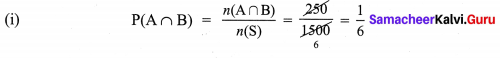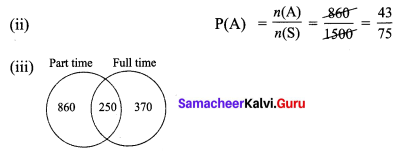Total families n(S) = 1500
No. of families have maids = 860 + 250 + 370 = 1480
No. of families do not have maids = 1500 – 1480
n( A) = 20